# Time Serie - Vizualization

Most of the Time serie database have also a visualization tool.

75% of business graphs display time series data.

The visual representation of the full year with four seasons can be seen:

• as a box,
• as a straight line
• or as a circle.

The visual mapping of time may be different across individuals.

## List

### Ggplot

• Request Time Histogram Diagram
``````# Transform the text as a POSIXct type
end_ts_time=as.POSIXct(res_succes\$END_TS, format="%Y-%m-%d %H:%M:%S", tz="UTC")
# Show it (User name is the thread name)
ggplotly(ggplot(res_succes, aes(x=end_ts_time, fill=factor(res_succes\$USER_NAME)) )
+ geom_histogram() # by default the value is stacked by fill (position="stack")
+ scale_x_datetime()
+ labs(title="Request Frequency Diagram", fill="Threads", x="Request End Time", y="Request Count"))```
```
• Request Time Frequency Diagram
``````end_ts_time=as.POSIXct(res_succes\$END_TS, format="%Y-%m-%d %H:%M:%S", tz="UTC")
ggplotly(ggplot(res_succes, aes(x=end_ts_time, color=factor(res_succes\$USER_NAME)) )
+ geom_freqpoly(binwidth = 30)
+ scale_x_datetime()
+ labs(title="Request Frequency Diagram", color="Threads", x="Request End Time", y="Request Count"))```
```

### Library

Application Name Technology Store
Cubism D3 - Javascript written by Mike Bostock showing horizon charts Only Viz
Grafana Only Viz
Graphite Store and Viz
Rickshaw Time Serie Built on d3.js Only Viz (Demo)
http://dygraphs.com/ Time Serie specialized Used by Netdata Demo

## Documentation / Reference

Discover More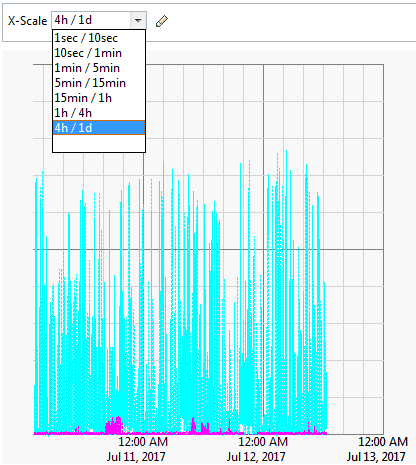Application Metrics (Perfcounter | Performance Metrics | Operational data | Monitoring )

Application Metrics (Perfcounter | Performance Metrics | Operational data | Monitoring ) This section is about the collection and calculation of metrics in the monitoring context. These (counter|numbers)...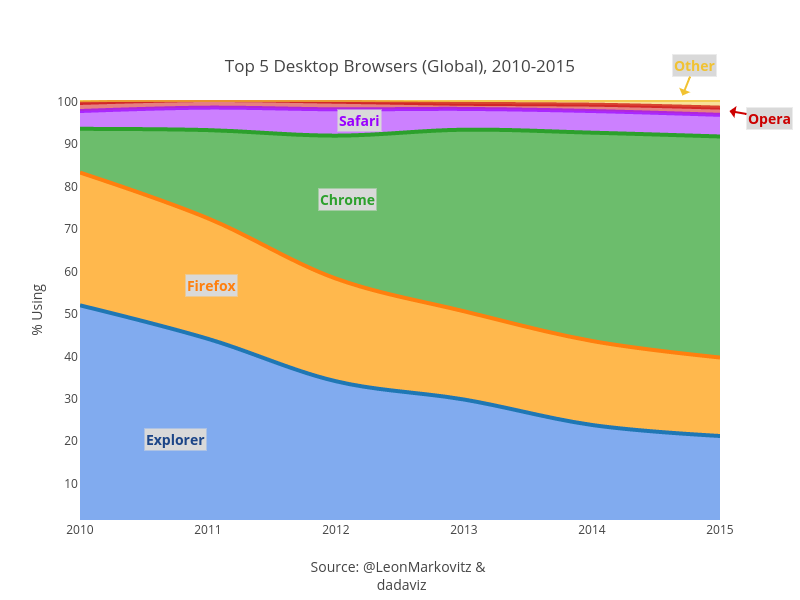Data Visualisation - Area Chart

An area chart shows the trend of the contribution of: each value over time or by category. It is basically a line graph for which the regions between lines are filled in. Regions stack, adding...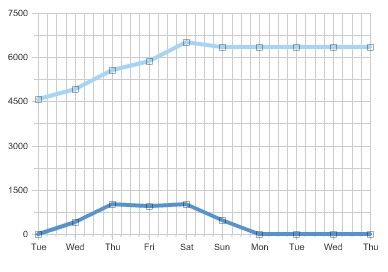Data Visualization - Line Chart

Line chart shows quantities: over time. time series or by category. Line graphs are useful for showing: trends over time. deviations between values. Can be used to plot multiple (variables|measures)...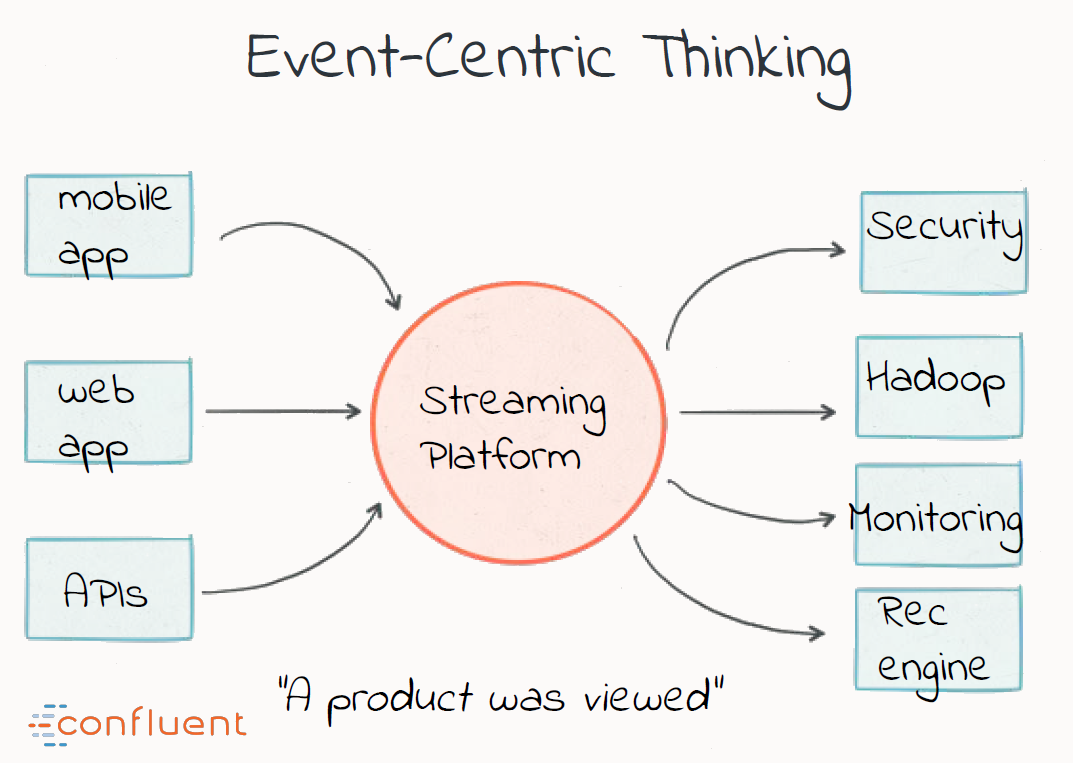Streaming Visualization

Streaming implied the notion of time and most of the streaming visualization are time series visualization where the origin of the x axis is moving at each refresh interval (ie the data below the origin...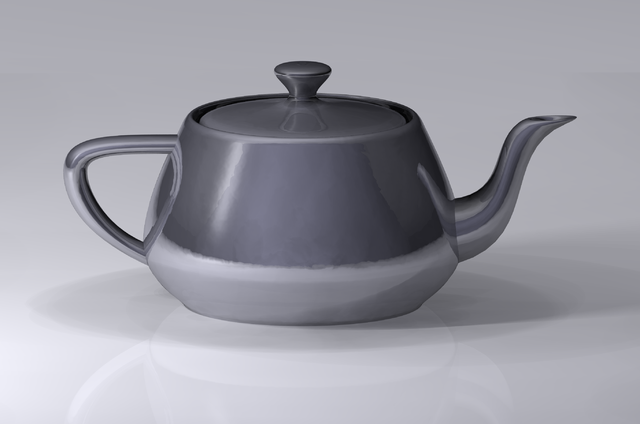Viz - Control Chart (Shewhart)

The purpose of control charts is to allow simple detection of events that are indicative of actual process change. Control charts attempt to differentiate “assignable” (“special”) sources of variation...